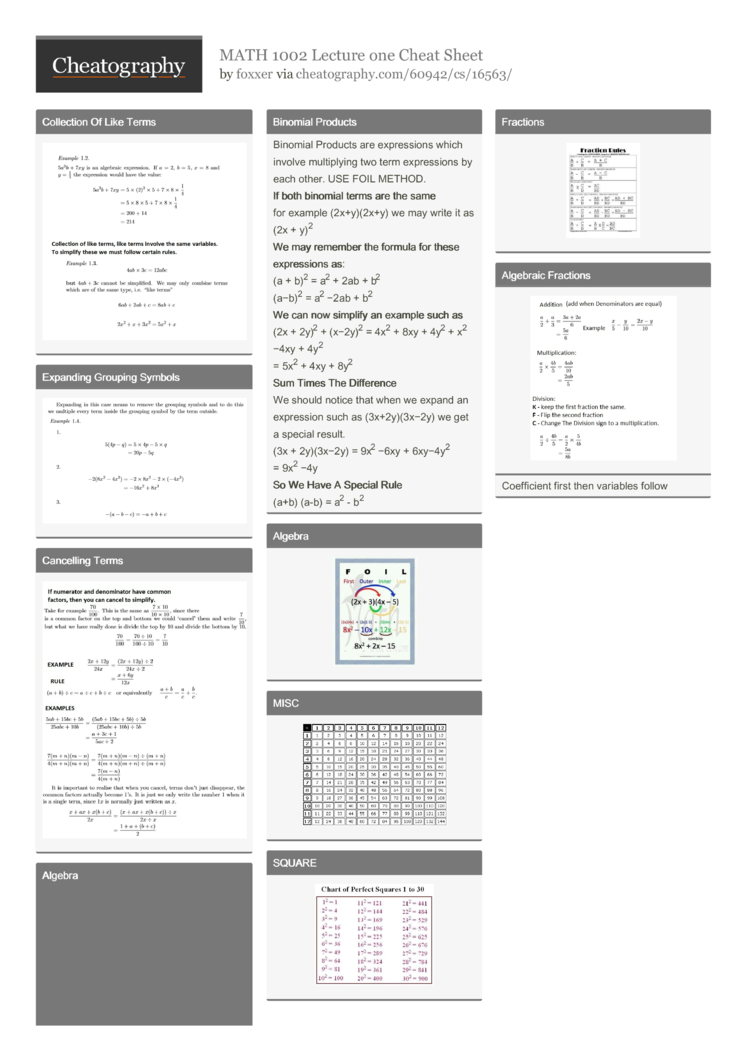MATH 1002 Lecture one Cheat Sheet by foxxer

Coll­ection Of Like TermsExpa­nding Grouping SymbolsCanc­elling TermsBinomial Products

 Binomial Products are expres­sions which involve multip­lying two term expres­sions by each other. USE FOIL METHOD. If both binomial terms are the same for example (2x+y)­(2x+y) we may write it as (2x + y)2 We may remember the formula for these expres­sions as: (a + b)2 = a2 + 2ab + b2 (a−b)2 = a2 −2ab + b2 We can now simplify an example such as (2x + 2y)2 + (x−2y)2 = 4x2 + 8xy + 4y2 + x2 −4xy + 4y2 = 5x2 + 4xy + 8y2 Sum Times The Differ­ence We should notice that when we expand an expression such as (3x+2y­)(3­x−2y) we get a special result. (3x + 2y)(3x−2y) = 9x2 −6xy + 6xy−4y2 = 9x2 −4y So We Have A Special Rule (a+b) (a-b) = a2 - b2

Alge­braic Fracti­onsCoeffi­cient first then variables follow2 Pages
//media.cheatography.com/storage/thumb/foxxer_math-1002-lecture-one.750.jpg

PDF (recommended)